#以陣列 (Array) 為基礎的佇列 (Queue)

## 佇列的抽象資料結構

``````Q is a queue.

sub IsEmpty(Q): bool
(Optional) IsFull(Q): bool
(Optional) Size(Q): sz
sub Peek(Q): data
(Optional) PeekRear(Q): data
sub Enqueue(Q, data): void
sub Dequeue(Q): data
``````

## 佇列的公開界面

``````#ifndef QUEUE_H
#define QUEUE_H

#ifndef __cplusplus
#include <stdbool.h>
#endif

typedef struct queue_t queue_t;

#ifdef __cplusplus
extern "C" {
#endif

queue_t * queue_new(void);
void queue_delete(void *self);
bool queue_is_empty(const queue_t *self);
int queue_peek(const queue_t *self);
bool queue_enqueue(queue_t *self, int data);
int queue_dequeue(queue_t *self);

#ifdef __cplusplus
}
#endif

#endif  /* QUEUE_H */
``````

## 佇列的型態宣告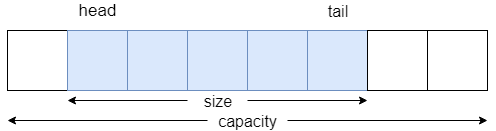``````typedef struct queue_t queue_t;

struct queue_t {
size_t size;
size_t capacity;
size_t tail;
int *elements;
};
``````

## 佇列的建構函式

``````queue_t * queue_new(void)
{
queue_t *q = malloc(sizeof(queue_t));
if (!q)
return q;

q->size = 0;
to test queue expansion. */
q->capacity = 2;

q->tail = 0;
q->elements = malloc(q->capacity * sizeof(int));
if (!(q->elements)) {
free(q);
q = NULL;
return q;
}

return q;
}
``````

## 佇列的解構函式

``````void queue_delete(void *self)
{
if (!self)
return;

int *elements = ((queue_t *) self)->elements;
if (elements)
free(((queue_t *) self)->elements);

free(self);
}
``````

## 檢查佇列是否為空

`IsEmpty(Q)` 的 C 程式碼如下：

``````bool queue_is_empty(const queue_t *self)
{
assert(self);

return self->size == 0;
}
``````

## 檢視佇列頭端的資料

`Peek(Q)` 的 C 程式碼如下：

``````int queue_peek(const queue_t *self)
{
assert(!queue_is_empty(self));

}
``````

## 將資料放入佇列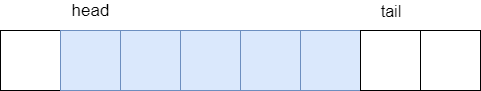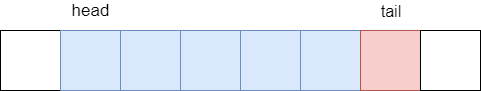``````bool queue_enqueue(queue_t *self, int data)
{
if (!queue_expand(self)) {
return false;
}

if (self->size == 0) {
self->elements[self->tail] = data;
self->size += 1;
return true;
}

self->tail = (self->tail + 1) % self->capacity;
self->elements[self->tail] = data;
self->size += 1;

return true;
}
``````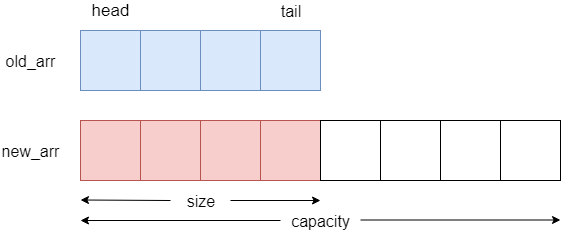``````static bool queue_expand(queue_t *self)
{
if (self->size < self->capacity) {
return true;
}

int *oldArr = self->elements;
self->capacity = self->size * 2;
int *newArr = malloc(self->capacity * sizeof(int));
if (!newArr) {
return false;
}

size_t sz = 0;
while (sz < self->size) {
newArr[i] = oldArr[j];

i = (i + 1) % self->capacity;
j = (j + 1) % self->size;
sz += 1;
}

self->elements = newArr;
self->tail = (self->head + self->size - 1) % self->capacity;
free(oldArr);

return true;
}
``````

## 將資料移出佇列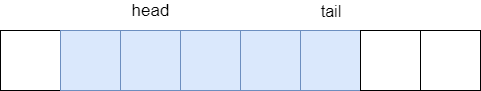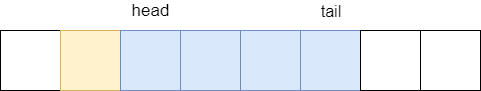``````int queue_dequeue(queue_t *self)
{
assert(!queue_is_empty(self));

self->size -= 1;

return popped;
}
``````

## 在演算法上的效率

• `IsEmpty`: `O(1)`
• `Peek`: `O(1)`
• `Enqueue`: amortized `O(1)`
• `Dequeue`: `O(1)`